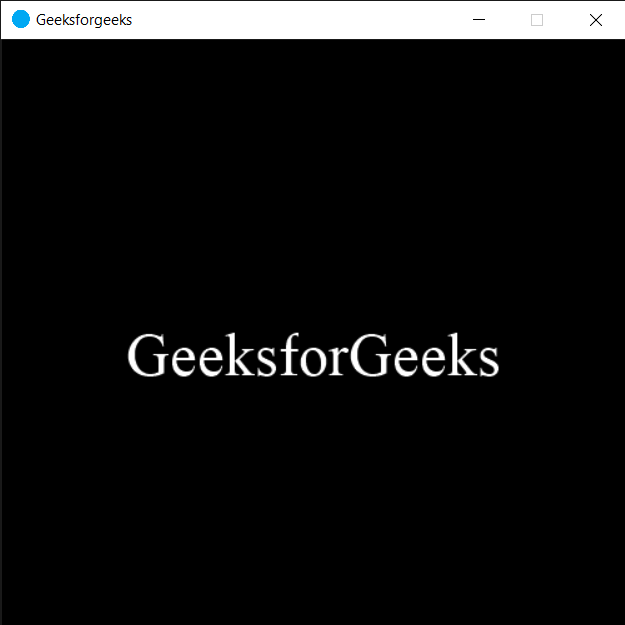Related Articles
PYGLET – Getting Window Context
• Last Updated : 22 Sep, 2020

In this article we will see how we can get the window context in PYGLET module in python. Pyglet is easy to use but powerful library for developing visually rich GUI applications like games, multimedia etc. A window is a “heavyweight” object occupying operating system resources. Windows may appear as floating regions or can be set to fill an entire screen (fullscreen). Window context is a OpenGL context attached to this window. It is a read only file.

We can create a window with the help of command given below

``` pyglet.window.Window(width, height, title)
```

In order to create window we use `context` attribute with the window object

Syntax : window.context

Argument : It takes no argument

Return : It returns Win32Context object

Below is the implementation

 `# importing pyglet module``import` `pyglet``import` `pyglet.window.key`` ` `# width of window``width ``=` `500`` ` `# height of window``height ``=` `500`` ` `# caption i.e title of the window``title ``=` `"Geeksforgeeks"`` ` `# creating a window``window ``=` `pyglet.window.Window(width, height, title)`` ` `# text ``text ``=` `"GeeksforGeeks"`` ` `# creating a label with font = times roman``# font size = 36``# aligning it to the centre``label ``=` `pyglet.text.Label(text,``                          ``font_name ``=``'Times New Roman'``,``                          ``font_size ``=` `36``,``                          ``x ``=` `window.width``/``/``2``, y ``=` `window.height``/``/``2``,``                          ``anchor_x ``=``'center'``, anchor_y ``=``'center'``)`` ` `new_label ``=` `pyglet.text.Label(text,``                          ``font_name ``=``'Times New Roman'``,``                          ``font_size ``=` `10``,``                          ``x ``=` `25``, y ``=` `25``)`` ` `# on draw event``@window``.event``def` `on_draw():    `` ` `     ` `    ``# clearing the window``    ``window.clear()``     ` `    ``# drawing the label on the window``    ``label.draw()`` ` `     ` `# key press event    ``@window``.event``def` `on_key_press(symbol, modifier):`` ` `    ``# key "C" get press``    ``if` `symbol ``=``=` `pyglet.window.key.C:``         ` `        ``# closing the window``        ``window.close()``     ` ` ` `# image for icon``img ``=` `image ``=` `pyglet.resource.image(``"logo.png"``)`` ` `# setting image as icon``window.set_icon(img)`` ` `# getting window context``value ``=` `window.context`` ` `# printing the value``print``(``"Window context : "``)``print``(value)`` ` `                ` `# start running the application``pyglet.app.run()`

Output :```Window context :
Win32Context()
```

Attention geek! Strengthen your foundations with the Python Programming Foundation Course and learn the basics.

To begin with, your interview preparations Enhance your Data Structures concepts with the Python DS Course.

My Personal Notes arrow_drop_up